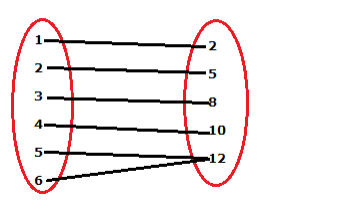# Let g = {(1, 2), (2, 5), (3, 8), (4, 10), (5, 12), (6, 12)}

Question:

Let g = {(1, 2), (2, 5), (3, 8), (4, 10), (5, 12), (6, 12)}. Is g a function? If yes, its domain range. If no, give reason.

Solution:

Given:

g = {(1, 2), (2, 5), (3, 8), (4, 10), (5, 12), (6, 12)}

We know that

A function ‘f’ from set A to set B is a correspondence (rule) which associates elements of set A to elements of set B such that:

(i) all elements of set $A$ are associated with an element in set $B$.

(ii) an element of set $A$ is associated with a unique element in set $B$Here, we observe that each element of the given set has appeared as the first component in one and only one ordered pair in ‘g.’ So, g is a function in the given set.

Dom (g) = 1, 2, 3, 4, 5, 6

Range (g) = 2, 5, 8, 10, 12